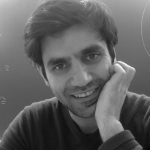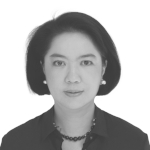# Set Theory in the United Kingdom 10

## University of Oxford Wednesday 14 June 2023

Set Theory in the United Kingdom is a research network in set theory that was formed in 2018 and has been funded by two Scheme 3 grants of the London Mathematical Society and an INI Network Support grant by the Isaac Newton Institute for Mathematical Sciences.

The members of the network are the Universities of Bristol, Cambridge, East Anglia, Leeds, Oxford, Warwick and University College London. The current coordinators are Andrew Brooke-Taylor (Leeds) and Benedikt Löwe (Cambridge).

STUK 10 is the tenth installment of the series and will take place in Classroom 3 in the Andrew Wiles Building of the Mathematical Institute of the University of Oxford (Woodstock Road, OX2 6GG; location information), Wednesday, 14 June 2023. The local organisers are Clara List, Emma Palmer, and Wojciech Wołoszyn.

Invited speakers:Joel David Hamkins (Notre Dame IN) Grigor Sargsyan (Sopot) Pimpen Vejjajiva (Bangkok).
11:00–12:00 Pimpen Vejjajiva (Bangkok): Infinite factorials and related cardinals Abstract. A factorial $$\mathfrak{m}!$$ is the cardinality of the set of permutations on a set which is of cardinality $$\mathfrak{m}$$. In this talk, I shall give relationships between some cardinals related to infinite factorials in the absence of the Axiom of Choice. Lunch Joel David Hamkins (Notre Dame IN): Set theory with abundant urelements Abstract. I shall speak on the surprising strength of the second-order reflection principle in the context of set theory with abundant urelements. The theory $$\mathsf{GBcU}$$ with the abundant urelement axiom and second-order reflection is bi-interpretable with a strengthening of $$\mathsf{KM}$$ with a supercompact cardinal. This is joint work with Bokai Yao. Coffee break Grigor Sargsyan (Sopot): Sealing theorems Abstract. We will explore different types of Sealing Theorems (generic absoluteness theorems), and will state a new one that seals the theory of $$\mathbf{L}(\mathrm{uB}, \mathbb{R})[\mathcal{C}]$$ where $$\mathrm{uB}$$ is the set of universally Baire sets and $$\mathcal{C}$$ is the club filter on $$\wp_{\omega_1}(\mathrm{uB})$$. This is joint work with Sandra Müller. Coffee break Informal presentations 16:00–16:15. Andrew Brooke-Taylor (Leeds). 16:15–16:45. Philipp Schlicht (Bristol).EPSRC EP/V521929/1